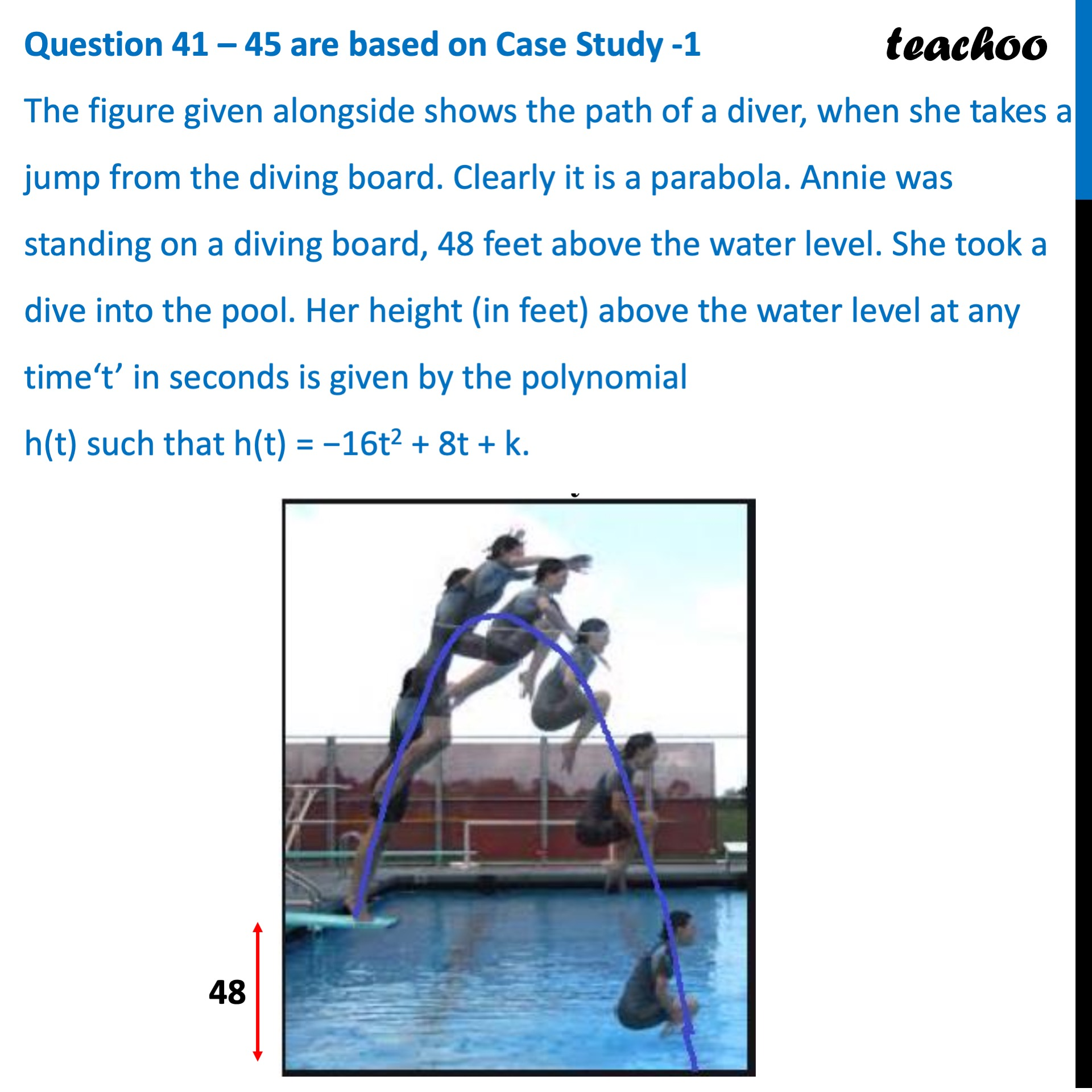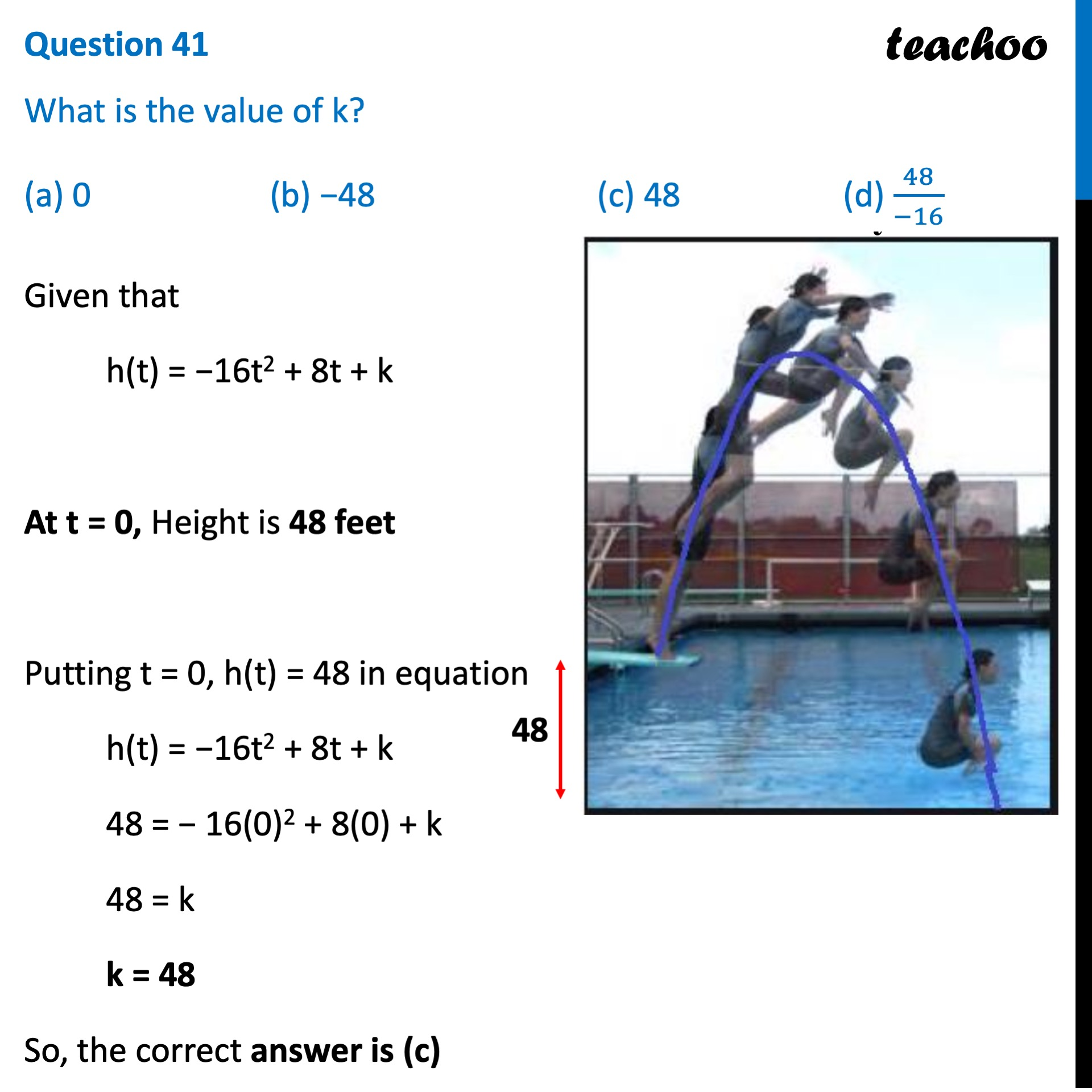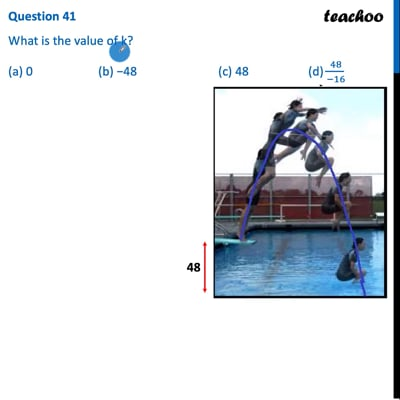CBSE Class 10 Sample Paper for 2022 Boards - Maths Standard [MCQ]

Class 10
Solutions of Sample Papers for Class 10 Boards

## The figure given alongside shows the path of a diver, when she takes a jump from the diving board. Clearly it is a parabola. Annie was standing on a diving board, 48 feet above the water level. She took a dive into the pool. Her height (in feet) above the water level at any time‘t’ in seconds is given by the polynomial h(t) such that h(t) = −16t2 + 8t + k.### Question 41 What is the value of k? (a) 0  (b) −48   (c) 48  (d) 48/(-16)(adsbygoogle = window.adsbygoogle || []).push({});This video is only available for Teachoo black users

Learn in your speed, with individual attention - Teachoo Maths 1-on-1 Class

### Transcript

Question 41 – 45 are based on Case Study -1 The figure given alongside shows the path of a diver, when she takes a jump from the diving board. Clearly it is a parabola. Annie was standing on a diving board, 48 feet above the water level. She took a dive into the pool. Her height (in feet) above the water level at any time‘t’ in seconds is given by the polynomial h(t) such that h(t) = −16t2 + 8t + k. Question 41 What is the value of k? (a) 0 (b) −48 (c) 48 (d) 48/(−16) Given that h(t) = −16t2 + 8t + k At t = 0, Height is 48 feet Putting t = 0, h(t) = 48 in equation h(t) = −16t2 + 8t + k 48 = − 16(0)2 + 8(0) + k 48 = k k = 48 So, the correct answer is (c)